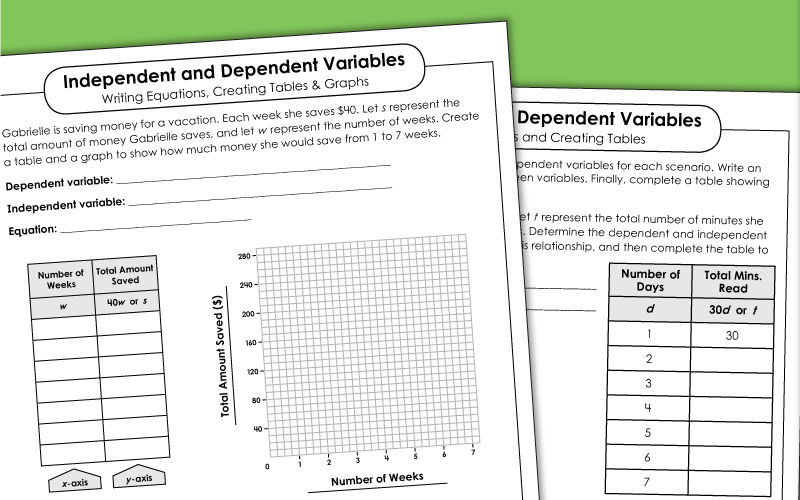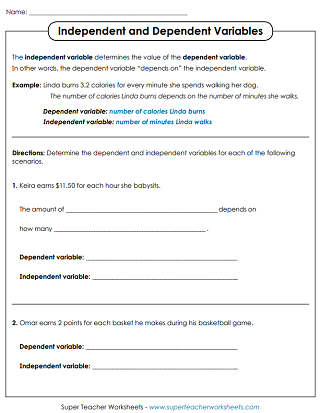# Dependent and Independent Variables

With these printable worksheets, students can review the differences between dependent and independent variables.## Dependent & Independent Variables Only

Read each sentence and determine which variables are independent and which ones are dependent.

## Dependent & Independent Variables w/ Equations

Determine the independent and dependent variables. Then write an equation for each
After finding the dependent and independent variables, students write and solve an equation for each scenario given.

## Independent & Dependent Variables w/ Equations, Tables, & Graphs

Figure out what the dependent and independent variable is for each word problem scenario. Then write an equation and complete a table for each.
Read the scenario. Then determine the dependent and independent variable. Write an equation. Then make a table and a graph.
Algebra & Pre-Algebra Worksheets

## Sample Worksheet ImagesMy Account
Site Information